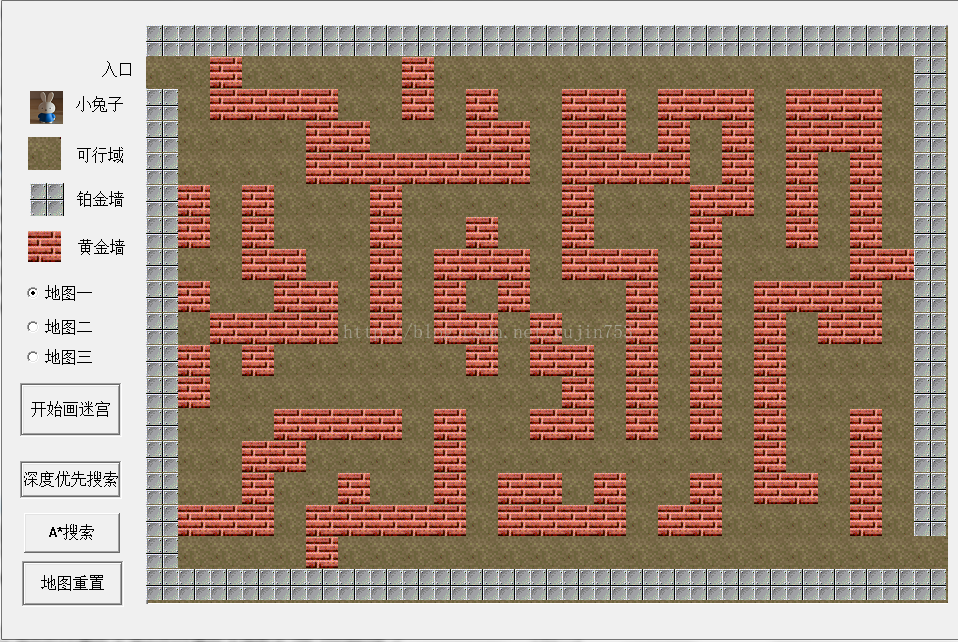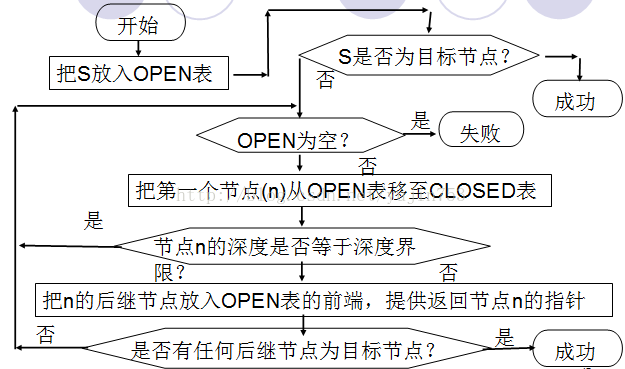# A*算法解决迷宫问题（DIY制作地图类似于小游戏，界面设计非常不错。）

2014/09/22 16:38

地图设计采用的是坦克大战中的地图设计图案。## 深度优先搜索算法实现

a) 一般不能保证找到最优解

b) 当深度限制不合理时，可能找不到解，可以将算法改为可变深度限制

c) 最坏情况时，搜索空间等同于穷举

d) 是一个通用的与问题无关的方法1) Push(S,Open) 将初始节点放入Open表中；

2) Judge(S) 判断S节点是不是目标节点，if（S为目标节点），那么找到目标节点；

3) While（判断Open表是否为空）

4) 将N的后继节点放入Open表中；

If（存在目标节点）

Else

## A*算法实现

A*算法是人工智能中的一种搜索算法，是一种启发式搜索算法，它不需遍历所有节点，只是利用包含问题启发式信息的评价函数对节点进行排序（Node Ordering），使搜索方向朝着最有可能找到目标并产生最优解的方向。它的独特之处是检查最短路径中每个可能的节点时引入了全局信息，对当前节点距终点的距离做出估计，并作为评价节点处于最短路线上的可能性的度量。

I．启发函数的确定

A*算法中引入了评估函数，评估函数如下：

f（n）=g（n）+h（n）

h（n）<=h（n）*

h（n）=|end.x – n.x|+ |end.y – n.y|

g（n）容易表示，即每走一步的代价是1，所以利用f（n）=g（n）+h（n）这种策略，我们可以不断地逼近目标点，从而找到问题的解。

II A*的主要实现步骤的伪码表示

1） OPEN:=(s), f(s):=g(s)+h(s);

2）LOOP: IF OPEN=( ) THEN EXIT(FAIL);

3） n:=FIRST(OPEN);

4）IF GOAL(n) THEN EXIT(SUCCESS);

6）EXPAND(n) →{mi},

IF f(n, mk)<f(mk) THEN f(mk):=f(n, mk),

IF f(n, ml)<f(ml,) THEN f(ml):=f(n, ml),

7, OPEN中的节点按f值从小到大排序；

8, GO LOOP；

# 编程实现

## 代码中数据结构设计

struct mark //定义迷宫内点的坐标类型

int x;

int y;

};

struct mark start,end; //start,end入口和出口的坐标

struct Element //栈元素，

{  int f;//估价函数

int g;//代价

int h;//启发函数

int x,y; //x行,y列

};

Element elem,e;

typedef struct LStack //链栈

{   Element elem;

struct LStack *next;

}*PLStack;

PLStack Open,Close;//OPEN表和CLOSE表用来储存链节点

## 函数框架实现

InitStack(Open); //用于初始化open 表

InitStack(Close); //用于初始化close表

Push(Open,elem); //表示初始节点elem进入open表

while(!StackEmpty(Open)&&Flag==0) //栈不为空 有路径可走

Pop(Open,elem); //将open表中的当前元素提出来

Push(Close,elem);//将从open表取出来的元素放入close表

if（判断elem节点的子节点是否可以走）判断上下左右{

if（elem_child不为目标节点）{

Push(Open,elem_child) 子节点进Open表，这里的push（）函数进入栈中，为有序进入，也就是说进栈是按顺序插入的。

}//if

else(该节点为目标节点){

Push(Close,elem_child)//目标节点进close表

Flag=1//将标志置为一表示已经找到了目标节点

End//找到了目标节点结束

}//else

}//if

}//while

If（StackEmpty(Open)||Flag==0）

Printf（”迷宫没有解\n”）

## 核心函数代码

int InitStack(PLStack &S) //初始化链栈，Open表和Close表初始化

{

S=NULL;

return 1;

}

int StackEmpty(PLStack S)//判断栈是否为空

if(S==NULL)

return 1;

else

return 0;

}

int Push1(PLStack &S, Element e)//压入新数据元素

PLStack p;

PLStack q,t;

p=(PLStack)malloc(sizeof(LStack));

p->elem.g=deep;//表示深度

//估价函数即使用曼哈顿距离表示

p->elem.h=abs(end.x-e.x)+abs(end.y-e.y);

p->elem.f=p->elem.h+p->elem.g;

p->elem.x=e.x;

p->elem.y=e.y;

p->next=NULL;

if(NULL==S||p->elem.f<=S->elem.f)

{

p->next=S;

S=p;

//return 1;

}

else

{

q=S;

t=q->next;

while(t!=NULL)

{

if(p->elem.f>t->elem.f)

{

q=t;

t=t->next;

}

else

break;

}

p->next=t;

q->next=p;

}

return 1;

int Pop(PLStack &S,Element &e) //栈顶元素出栈

PLStack p;

if(!StackEmpty(S))

e=S->elem;

p=S;

S=S->next;

free(p);

return 1;

else

return 0;

}

## 界面设计

界面设计非常友好、清晰、表达明确、功能集中、操作简单。

HWND hmap;

HDC hm;

hmap = GetDlgItem(hwnd,IDC_MAP);

hm = GetDC(hmap);

BitBlt(hm,x,y,p_Lsize,p_Hsize,h,0,0,SRCCOPY);

## 运行结果分析

 地图 所走步数 深度优先搜索 A*搜索 地图一 178 90 地图二 207 87 地图三 218 82

0
1 收藏

### 作者的其它热门文章0 评论
1 收藏
0